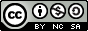Want to share your content on R-bloggers? click here if you have a blog, or here if you don't.

Recently I was tasked with evaluating and most importantly removing analytical variance form a longitudinal metabolomic analysis carried out over a few years and including >2,5000 measurements for >5,000 patients. Even using state-of-the-art analytical instruments and techniques long term biological studies are plagued with unwanted trends which are unrelated to the original experimental design and stem from analytical sources of variance (added noise by the process of measurement). Below is an example of a metabolomic measurement with and without analytical variance.The noise pattern can be estimated based on replicated measurements of quality control samples embedded at a ratio of 1:10 within the larger experimental design. The process of data normalization is used to remove analytical noise from biological signal on a variable specific basis. At the bottom of this post, you can find an in-depth presentation of how data quality can be estimated and a comparison of many common data normalization approaches. From my analysis I concluded that a relatively simple LOESS normalization is a very powerful method for removal of analytical variance. While LOESS (or LOWESS), locally weighted scatterplot smoothing, is a relatively simple approach to implement; great care has to be taken when optimizing each variable-specific model.

In particular, the span parameter or alpha controls the degree of smoothing and is a major determinant if the model  (calculated from repeated measures) is underfit, just right or overfit with regards to correcting analytical noise in samples. Below is a visualization of the effect of the span parameter on the model fit.One method to estimate the appropriate span parameter is to use cross-validation with quality control samples. Having identified an appropriate span, a LOESS model can be generated from repeated measures data (black points) and is used to remove the analytical noise from all samples (red points).Having done this we can now evaluate the effect of removing analytical noise from quality control samples (QCs, training data, black points above) and samples (test data, red points) by calculating the relative standard deviation of the measured variable (standard deviation/mean *100). In the case of the single analyte, ornithine, we can see (above) that the LOESS normalization will reduce the overall analytical noise to a large degree. However we can not expect that the performance for the training data (noise only) will converge with that of the test set, which contains both noise and true biological signal.

In addition to evaluating the normalization specific removal of analytical noise on a univariate level we can also use principal components analysis (PCA) to evaluate this for all variables simultaneously. Below is an example of the PCA scores for non-normalized and LOESS normalized data.We can clearly see that the two largest modes of variance in the raw data explain differences in when the samples were analyzed, which is termed batch effects. Batch effects can mask true biological variability, and one goal of normalizations is to remove them, which we can see is accomplished in the LOESS normalized data (above right).

However be forewarned, proper model validation is critical to avoiding over-fitting and producing complete nonsense.In case you are interested the full analysis and presentation can be found below as well as the majority of the R code used for the analysis and visualizations.